This notebook demos clustering in R.The data set is taken from http://people.sc.fsu.edu/~jburkardt/datasets/hartigan/hartigan.html which is a collection of data sets which can be used for clustering practice. The data set is file number 40 in the list and contains measurements for pH, HCO3 (bicarbonate) and CO2 (carbon dioxide) of cerebrospinal fluid and blood of 40 acidosis patients.Acidosis is a condition where the bodily fluids are too acidic, typically resulting from metabolic causes but may also be caused by respiratory difficulties.

First lets load the necessary packages and take a look at the data.

``````library (NbClust)
library (cluster)
library (clustertend)
library (factoextra)``````
``## Loading required package: ggplot2``
``## Welcome! Related Books: `Practical Guide To Cluster Analysis in R` at https://goo.gl/13EFCZ``
``````library (fpc)
library (clValid)
setwd("~/degreesofbelief/clustering")
data``````
``````##      X1   X2   X3   X4   X5   X6
## 1  39.8 38.0 22.2 23.2 38.8 36.5
## 2  53.7 37.2 18.7 18.5 45.1 28.3
## 3  47.3 39.8 23.3 22.1 48.2 36.4
## 4  41.7 37.6 22.8 22.3 41.6 34.6
## 5  44.7 38.5 24.8 24.4 48.5 38.8
## 6  47.9 39.8 22.0 23.3 46.2 38.5
## 7  48.4 36.7 21.0 21.3 44.5 32.6
## 8  48.4 35.1 23.9 24.0 50.6 35.0
## 9  48.4 45.7 18.6 14.9 39.4 28.8
## 10 41.7 81.3  9.8  4.2 17.8 12.9
## 11 46.2 42.7 15.5 15.0 31.3 26.8
## 12 48.4 42.2 19.6 18.7 41.6 32.6
## 13 49.6 55.0 14.6 11.3 31.8 25.7
## 14 47.3 59.4 10.4  7.5 21.5 18.6
## 15 42.7 49.0 15.3  9.5 26.9 19.0
## 16 38.5 47.9 13.7  9.4 23.0 18.7
## 17 46.2 36.1 23.2 27.3 46.9 39.8
## 18 51.3 39.6 23.1 26.8 52.3 43.3
## 19 49.0 40.1 18.9 20.0 40.4 32.4
## 20 46.0 41.4 18.9 20.0 44.8 40.1
## 21 50.9 40.8 23.3 25.5 52.0 42.3
## 22 50.0 41.4 24.6 25.2 53.8 42.3
## 23 49.0 39.5 24.5 26.4 52.4 42.3
## 24 49.4 40.9 22.9 28.5 53.1 47.2
## 25 47.2 38.5 27.2 26.4 54.9 40.9
## 26 47.7 38.0 26.2 29.2 53.5 44.6
## 27 49.0 41.9 27.6 28.9 58.0 49.0
## 28 53.1 39.4 26.2 33.0 59.9 52.2
## 29 52.5 53.8 29.4 32.0 66.2 56.9
## 30 51.3 41.7 28.4 31.1 62.6 52.4
## 31 52.7 43.8 30.4 34.6 69.0 61.4
## 32 48.2 38.6 29.4 34.0 60.6 52.6
## 33 42.7 37.2 20.7 18.6 38.6 29.2
## 34 44.2 33.9 20.7 19.1 40.3 26.8
## 35 43.6 35.5 21.9 21.8 41.7 32.2
## 36 49.0 33.9 22.4 23.3 46.9 30.6
## 37 54.9 33.8 22.9 34.8 45.1 32.1
## 38 46.6 37.3 22.5 24.9 44.8 37.4
## 39 47.5 36.4 22.3 22.9 45.4 33.3
## 40 44.3 32.8 22.8 24.8 42.8 32.5``````

Lets add column names based on the information provided with the dataset. We have measurements for 40 patients on 6 variables. If we do a quick plot of pairwise comparisons for each of the variables, tt is apparent that the are quite strong linear relationships between some of the variables for example CO2 in blood and cerebrospinal fluid.

``````colnames (data) <- c("pHCerbero", "pHBlood", "HCO3Cerebro", "HCO3Blood", "CO2Cerbero", "CO2Blood")
plot (data)``````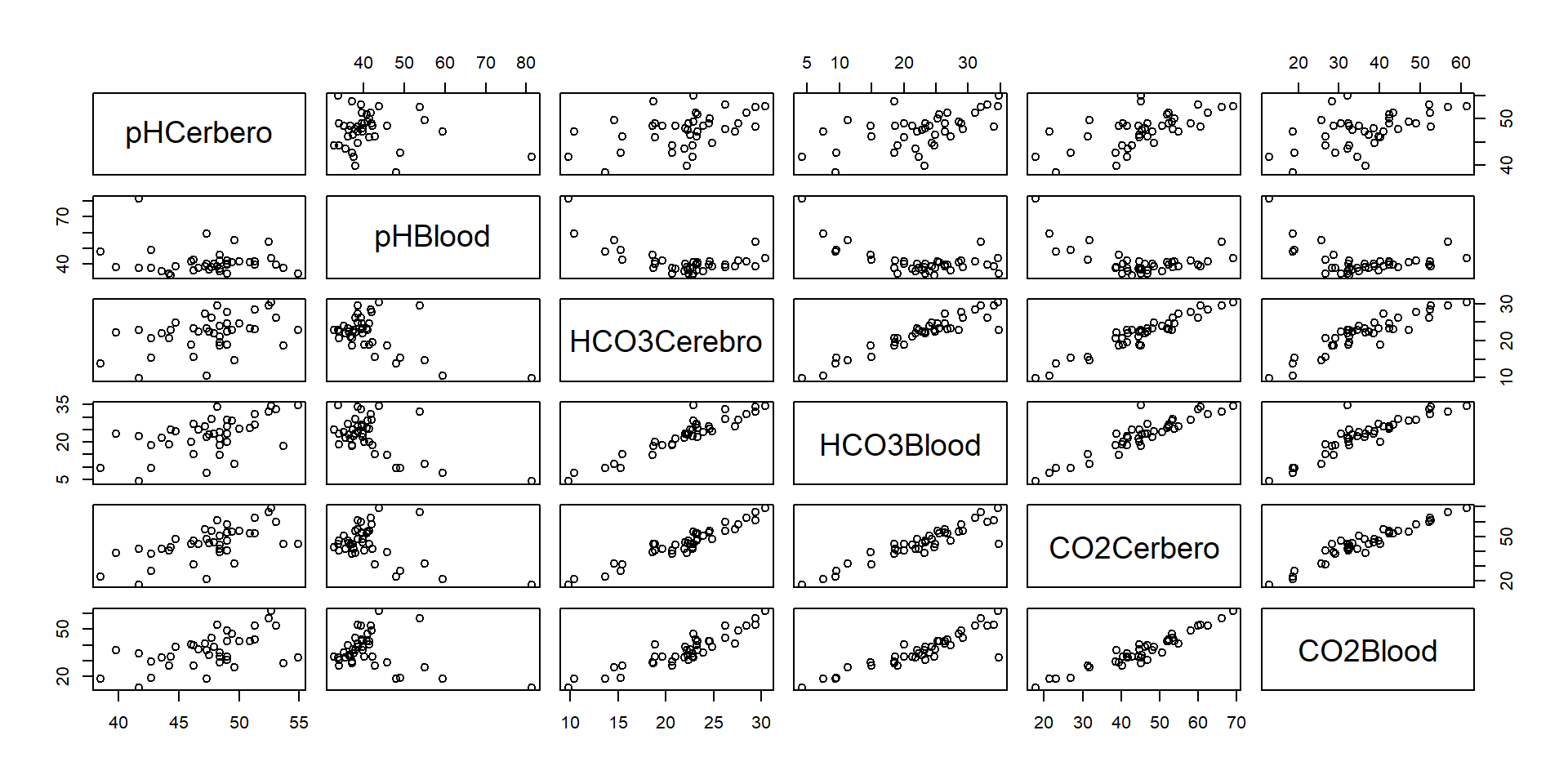I am going to use k means clustering for this analysis. The cluster analysis comprises the following steps…

1.Assess clustering tendency of the data
2.Estimate number of clusters
3.Run Clustering Algorithm
4.Assess Validity of Clustering Results

### Clustering Tendency

There are various ways to assess the clustering tendency of the data. Hopkins Test is used to test the probability that the data is generated by a uniform random distribution and is often used as a proxy measure for clustering tendency.

It works by selecting a sample from the data, the size of which is specified by the analyst. For each point find its distance to its nearest neighbour. Then the algorithm generates a simulated data set from a uniform random distribution and finds the distance from points in this data set from their nearest neighbours. To calculate the Hopkins statistic you divide the average nearest neighbour distance in the random dataset by the average nearest neighbour distance in the random dataset plus the average nearest neighbour distance in the actual dataset. Values close to 0.5 indicate high degree of spatial randomness.

``hopkins <- hopkins (data, n=nrow(data)-1)``

The result of the Hopkins Test is 0.1839671 which is well below the 0.5 threshold and therefore indicates the data is clusterable.

We can also use a visual method to assess clustering tendency. Visual Assessment of Clustering Tendency works by computing the dissimilarity matrix of objects in the data set (using Euclidean distance). Reorder the matrix so that similar objects are close to one another.

``````fviz_dist(dist(data), show_labels = FALSE)+
labs(title = "acidosis")``````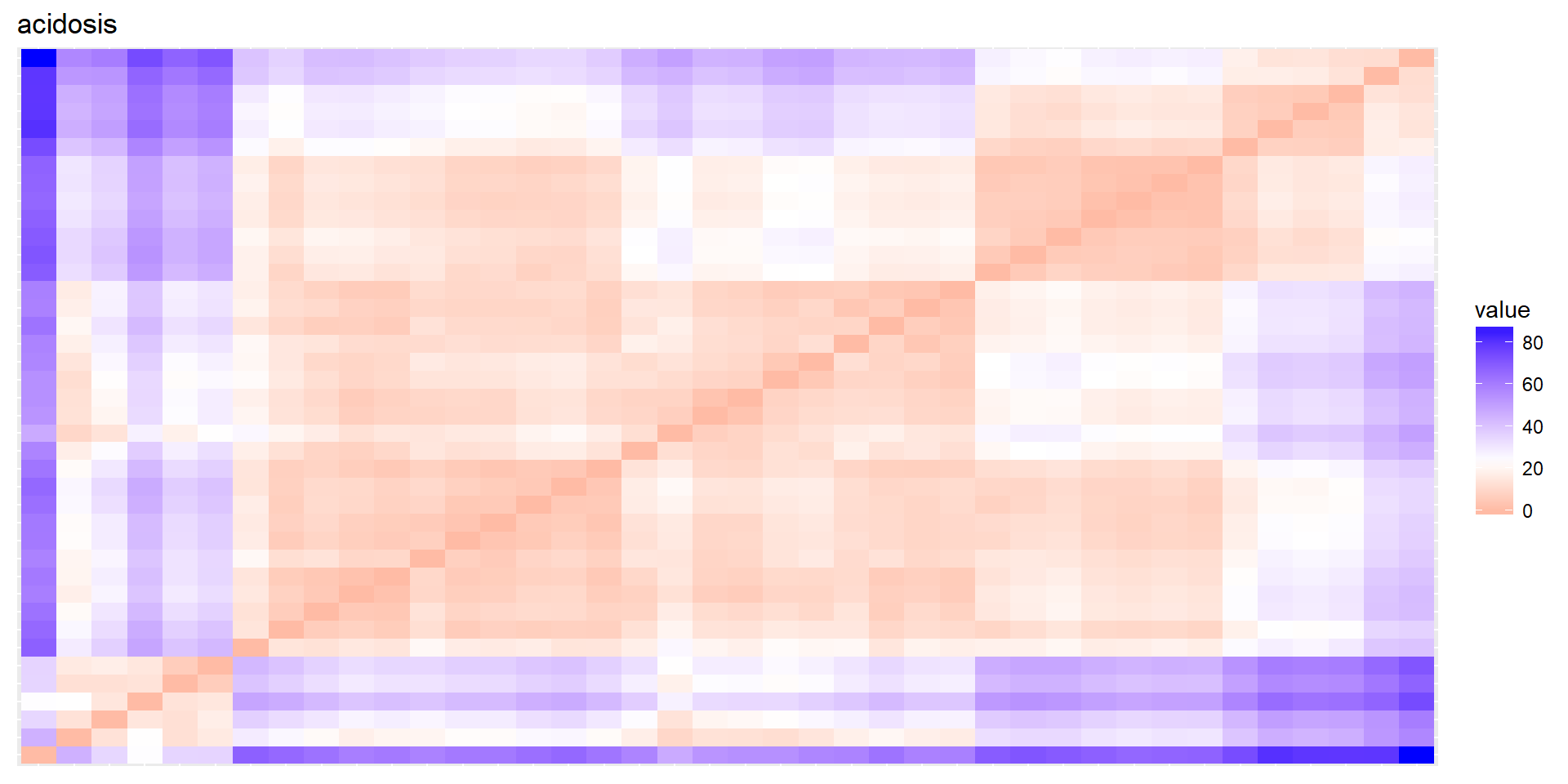Above is the ordered dissimilarity image of the acidosis data. This can be compared to one generated from random data below.

``````random <- replicate(6, sample (1:60, 40, rep=TRUE))
fviz_dist(dist(random), show_labels = FALSE)+
labs(title = "random_data")``````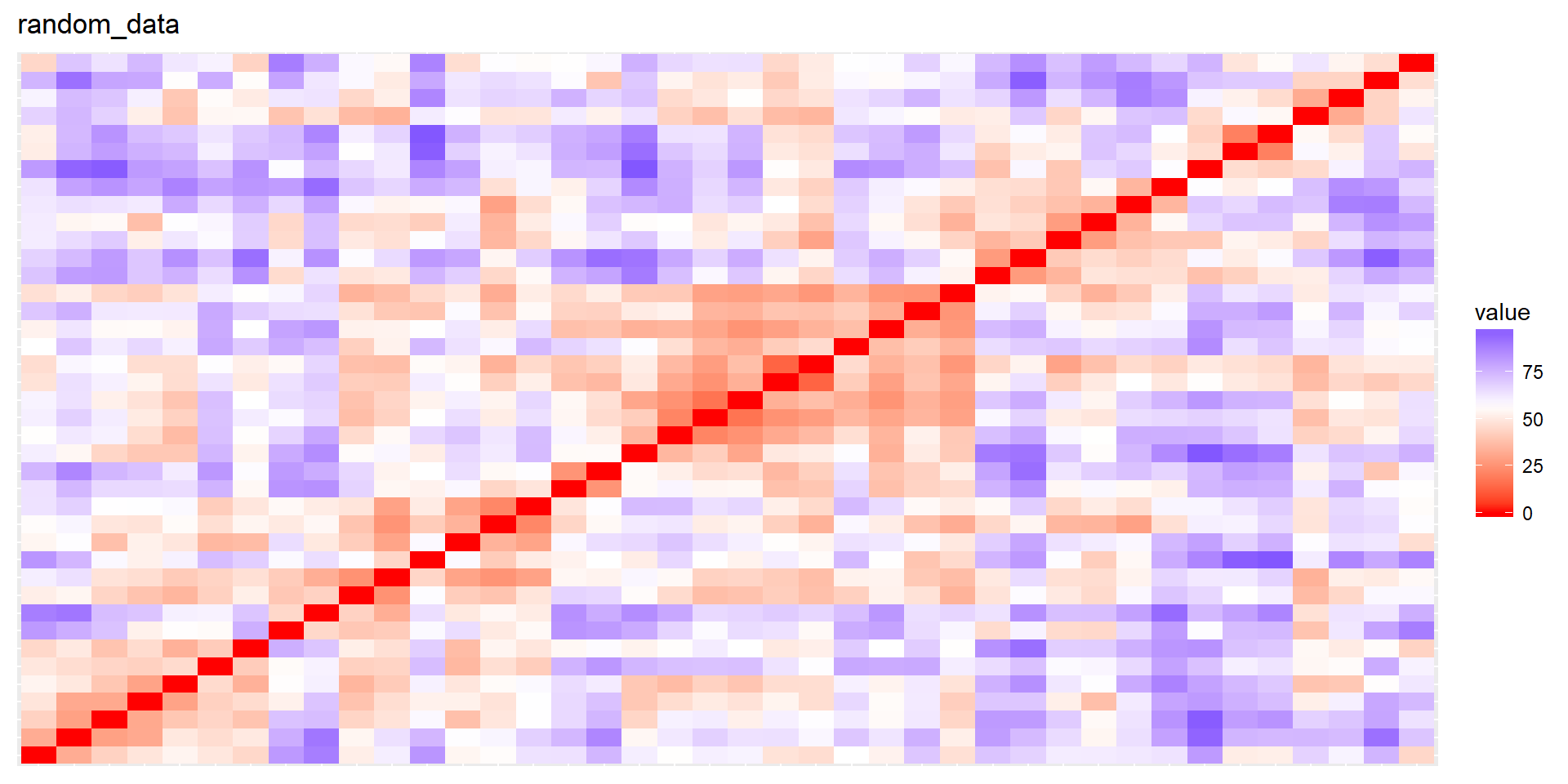It is apparent that there are patterns in the ordered dissimilarity image for the acidosis data but not for the random data.

### Estimate number of Clusters

We have shown that this dataset is clusterable. We are going to use k means algorithm to cluster the data and this requires us to specify how many clusters the dataset has so the next thing to do is to estimate this.

``fviz_nbclust(data, pam, method = "silhouette")+ theme_classic()``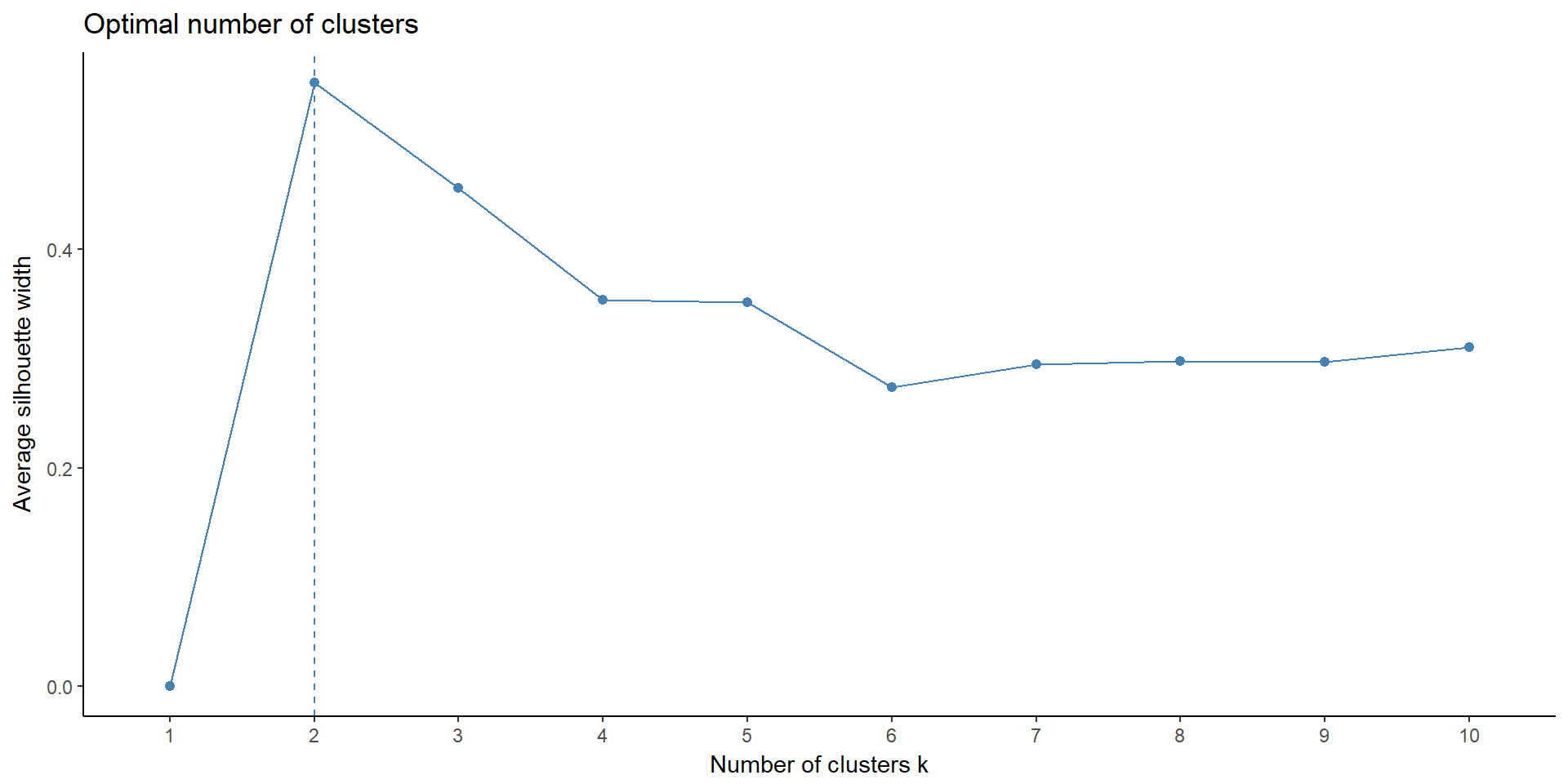Above is the silhouette plot of the data. The silhouette co efficient estimates the average distance between clusters. The silhouette plot shows the silhouette co efficient over values of k ranging from 1 to 10. This plot shows the highest average silhouette co-efficient occurring when k=2.

The gap statistic compares intra cluster variation for different values of k with expected intra cluster variation under null distribution. Ideally we should be choosing the value of k which maximizes the gap statistic however in real world datasets where clusters are not so well defined it may be more parsimonious to choose the k value to be the one where the rate of increase of the statistic begins to slow down (i.e. value lowest value of k that is greater than or equal to the value of k+1 minus the standard error).

``fviz_nbclust(data, pam, method = "gap_stat")``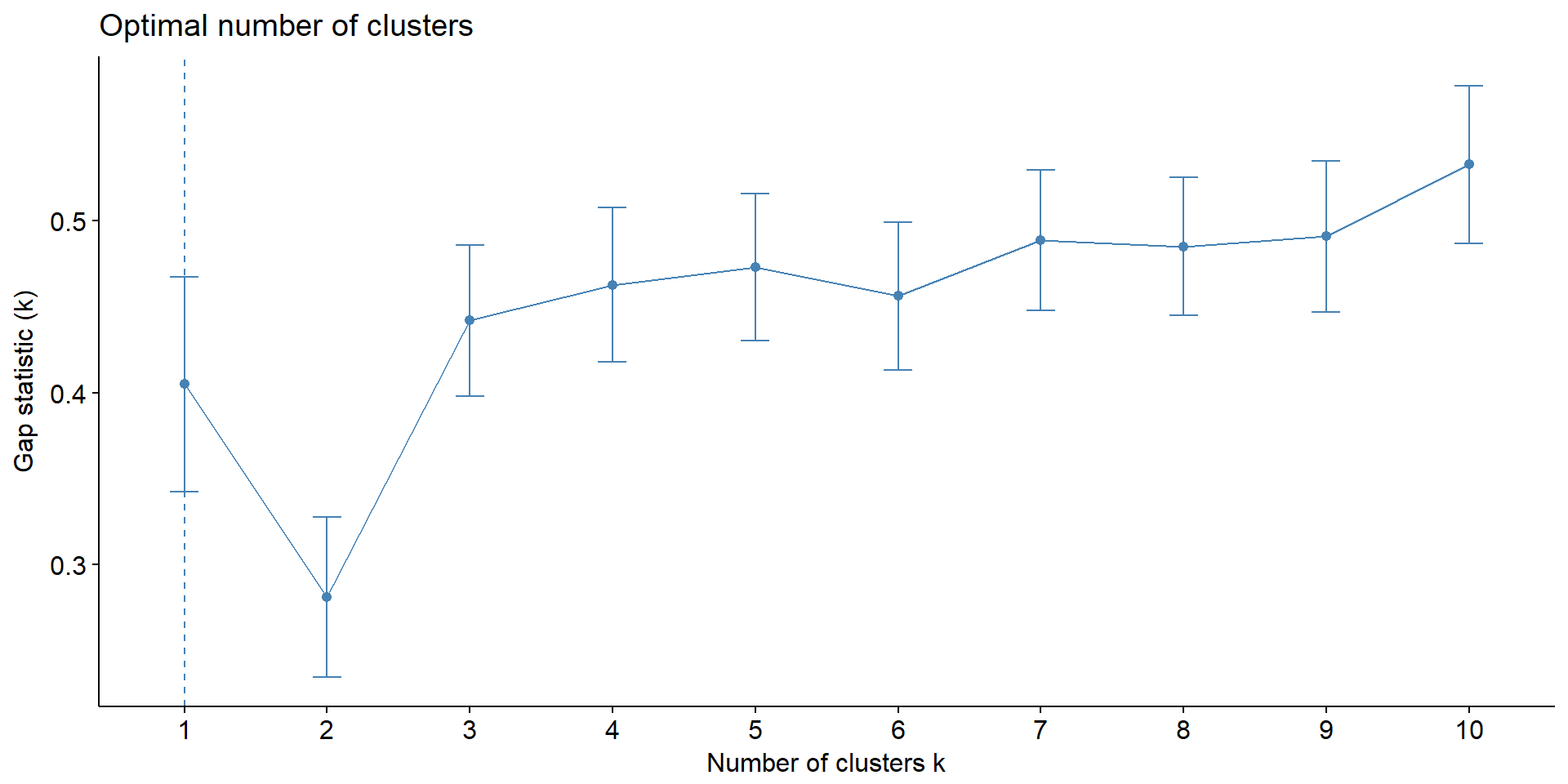In the graph above athis appears to occur when k=3.

We can also plot the first two principal components of the dataset to visually inspect how many clusters may be in the data set.

``fviz_pca_ind(prcomp(data), title = "PCA - Acidosis Data", palette = "jco", geom = "point", ggtheme = theme_classic(), legend = "bottom")``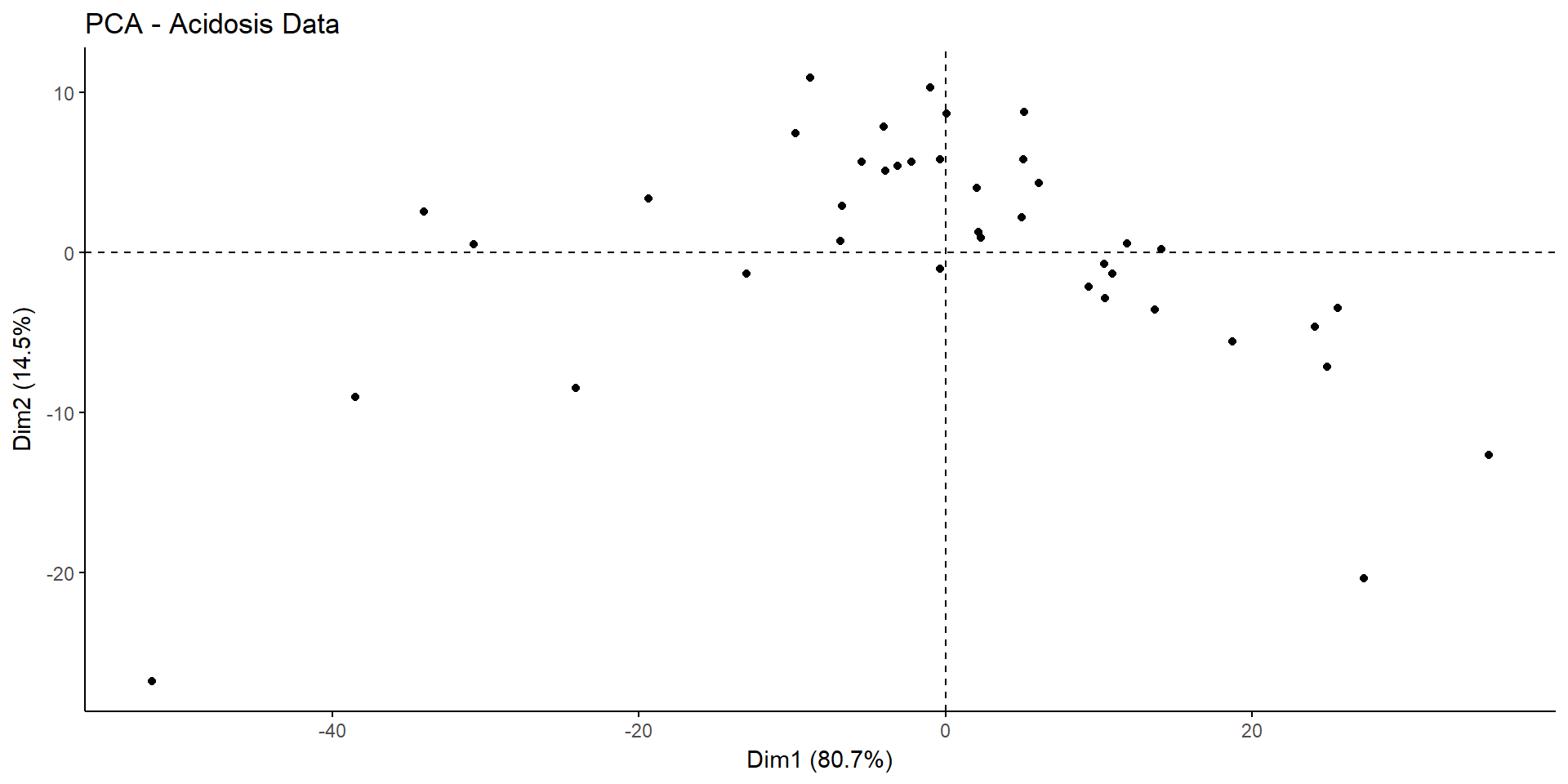Its hard to say for sure how many clusters are in the dataset just from inspecting the graph of the first two principal components. Two or three clusters looks right.

Next we can run NbClust which computes up to 30 indices for determining the optimum number of clusters in a dataset and then takes a majority vote among them to see which is the optimum number of clusters.

``clusternum <- NbClust ((data), distance="euclidean", method="kmeans")``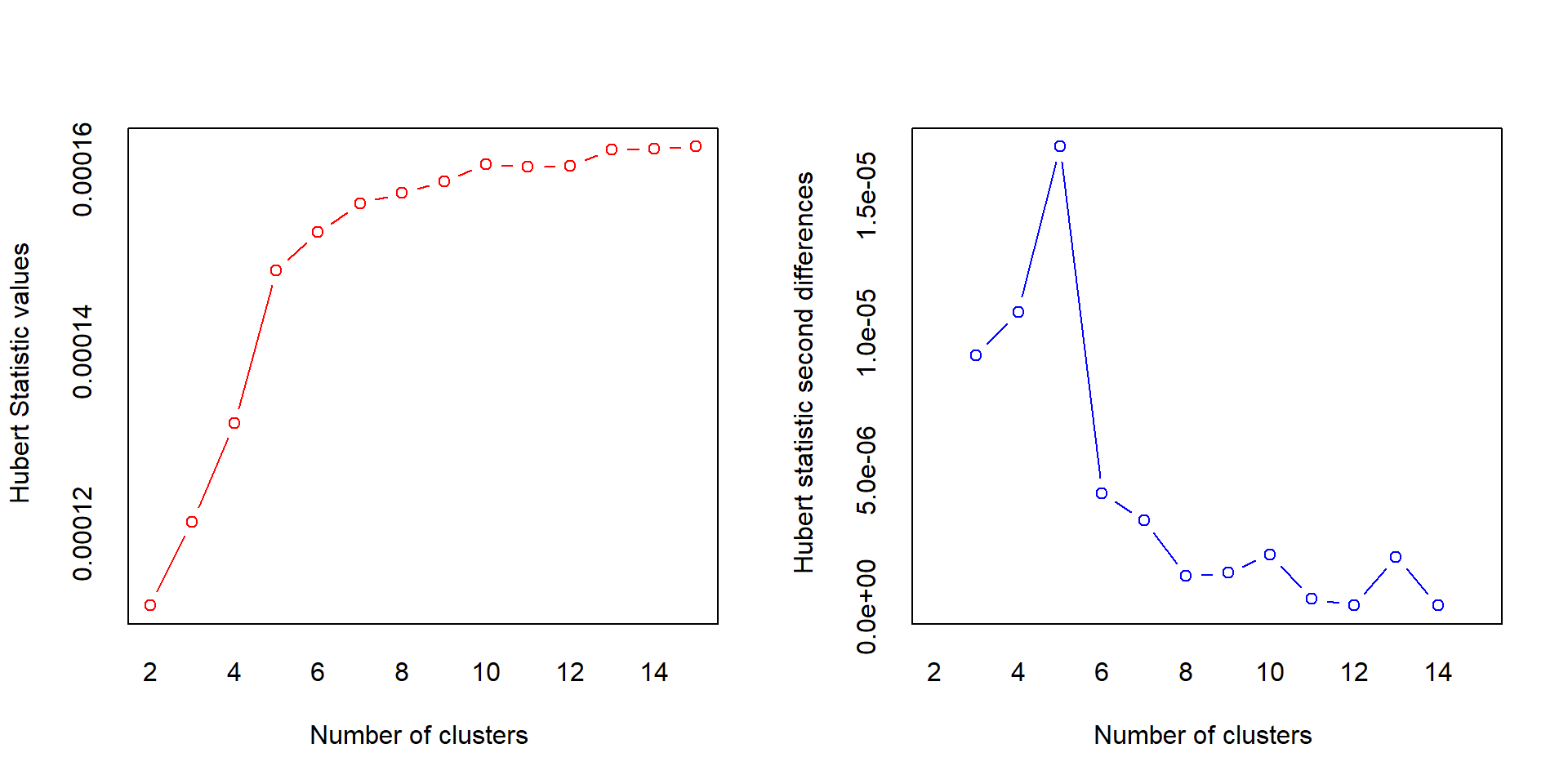``````## *** : The Hubert index is a graphical method of determining the number of clusters.
##                 In the plot of Hubert index, we seek a significant knee that corresponds to a
##                 significant increase of the value of the measure i.e the significant peak in Hubert
##                 index second differences plot.
## ``````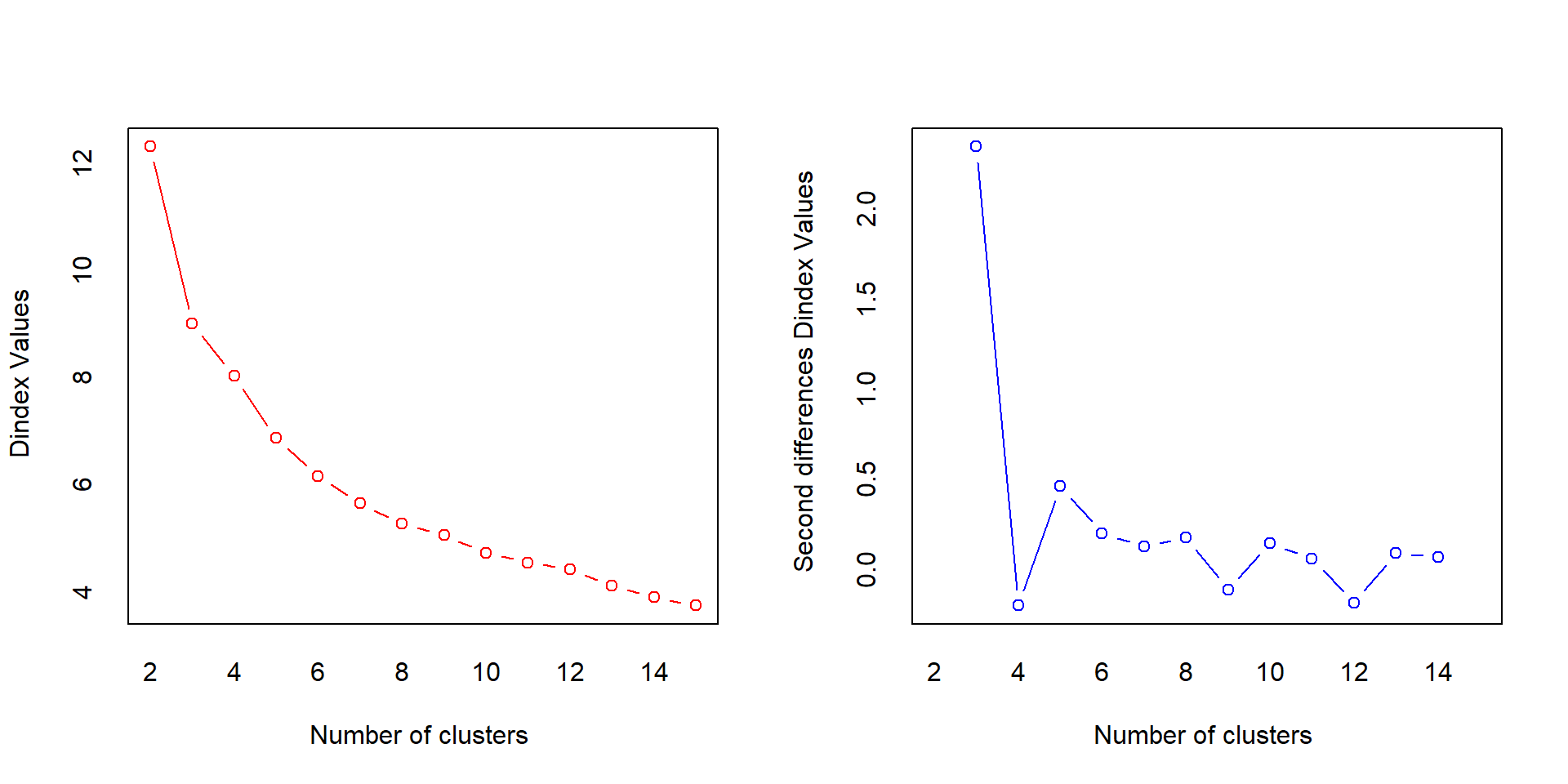``````## *** : The D index is a graphical method of determining the number of clusters.
##                 In the plot of D index, we seek a significant knee (the significant peak in Dindex
##                 second differences plot) that corresponds to a significant increase of the value of
##                 the measure.
##
## *******************************************************************
## * Among all indices:
## * 5 proposed 2 as the best number of clusters
## * 9 proposed 3 as the best number of clusters
## * 5 proposed 4 as the best number of clusters
## * 1 proposed 5 as the best number of clusters
## * 1 proposed 8 as the best number of clusters
## * 2 proposed 10 as the best number of clusters
## * 1 proposed 15 as the best number of clusters
##
##                    ***** Conclusion *****
##
## * According to the majority rule, the best number of clusters is  3
##
##
## *******************************************************************``````
• Among all indices:
• 3 proposed 2 as the best number of clusters
• 12 proposed 3 as the best number of clusters
• 1 proposed 4 as the best number of clusters
• 1 proposed 6 as the best number of clusters
• 1 proposed 9 as the best number of clusters
• 2 proposed 14 as the best number of clusters
• 4 proposed 15 as the best number of clusters

``           ***** Conclusion *****                            ``
• According to the majority rule, the best number of clusters is 3

If you want to see exactly which indices proposed which cluster number then running clusternum\$All.index will give you the index names. Links to papers explaining how the indices work are in the help documentation for the function.

### Run Cluster Algorithm

NbClust indicates the best number of clusters is 3. I have used PAM (Partitioning Around Medoids) algorithm which is a more robust form of the k means algorithm and specified 3 clusters.

``pam.res3 <- pam(data, 3,  metric = "euclidean", stand = FALSE)``

We can visualise the results. The plot shows the first two components of the data set and how the points cluster. The label indicates which row of the dataset the points relate to.

``````fviz_cluster(pam.res3, data = data, palette = c("#FC4E07", "#00AFBB", "#E7B800"), ellipse.type = "euclid",
star.plot = TRUE,
repel = TRUE,
ggtheme = theme_minimal() )``````